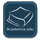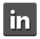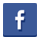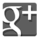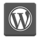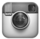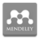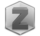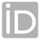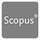You are in:Home/Courses

### Dr. Mohamed Husien Mohamed Eid :: Courses details:

 Number of courses : 35 Number of uploaded files for these courses from students : 0 Math IV-Mech-Aviation Institute Files(3) URL(-) Assignments(-) Exam(-)Math III-Comm-Aviation Institute Files(3) URL(-) Assignments(-) Exam(-)Math III-Aer-Aviation Institute Files(3) URL(-) Assignments(-) Exam(-)Math 6 - Credit Hours System - Control Files(3) URL(-) Assignments(-) Exam(-)Math 5 - Credit Hours System - Communications Files(5) URL(-) Assignments(-) Exam(-)Math 5 - Credit Hours System - Control Files(3) URL(-) Assignments(-) Exam(2)Math V-Pyramids Institute Files(-) URL(-) Assignments(-) Exam(1)Math IV-Aer-Aviation Institute Files(4) URL(-) Assignments(-) Exam(1)Math IV-Comm-Aviation Institute Files(5) URL(-) Assignments(-) Exam(1)Math 3 - Credit Hours System Files(5) URL(-) Assignments(-) Exam(6)Math 2 - Credit Hours System Files(4) URL(-) Assignments(-) Exam(9)Mathematics IV - MTI University-Eng. Files(2) URL(-) Assignments(-) Exam(1)Operations Research Files(1) URL(-) Assignments(-) Exam(5)Linear Algebra Files(1) URL(-) Assignments(-) Exam(7)Mathematics 1-B (Shoubra-Prep Year) Files(3) URL(-) Assignments(-) Exam(10)Mathematics 1-A (Shoubra-Prep Year) Files(6) URL(-) Assignments(-) Exam(17)Mathematics II (October Institute) Files(-) URL(-) Assignments(-) Exam(12)Mathematics I (October Institute) Files(1) URL(-) Assignments(-) Exam(12)Mathematics II (Pyramids Institute) Files(1) URL(-) Assignments(-) Exam(8)Mathematics I (Pyramids Institute) Files(3) URL(-) Assignments(-) Exam(9)Mathematics - Pharmacy - MTI University Files(3) URL(-) Assignments(-) Exam(24)Mathematics II (Eng. MTI University) Files(3) URL(-) Assignments(-) Exam(15)Mathematics I (Eng. MTI University) Files(2) URL(-) Assignments(-) Exam(10)Mathematics 1 (First year-Mech Eng-Production) Files(-) URL(-) Assignments(-) Exam(1)Mathematics 2-A (First year-Mech.Eng-Power) Files(-) URL(-) Assignments(-) Exam(1)Mathematics 3A (Second Year - M.Eng-Power) Files(-) URL(-) Assignments(-) Exam(2)Mathematics and N. Analysis (Second Year-Civil) Files(-) URL(-) Assignments(-) Exam(7)Mathematics B (Survying) Files(-) URL(-) Assignments(-) Exam(2)Mathematics A (Survying) Files(-) URL(-) Assignments(-) Exam(2)Mathematics 2-B (First year-E-Power) Files(-) URL(-) Assignments(-) Exam(2)Mathematics 2-A (First Year E.Power) Files(-) URL(-) Assignments(-) Exam(7)Mathematics 3B (Second Year-E-Comm) Files(1) URL(-) Assignments(-) Exam(2)Mathematics 2-B (First year-E-Power) Files(1) URL(-) Assignments(-) Exam(2)Math 4 - Credit Hours System Files(5) URL(-) Assignments(-) Exam(6)Math 1 - Credit Hours System Files(4) URL(-) Assignments(-) Exam(10)